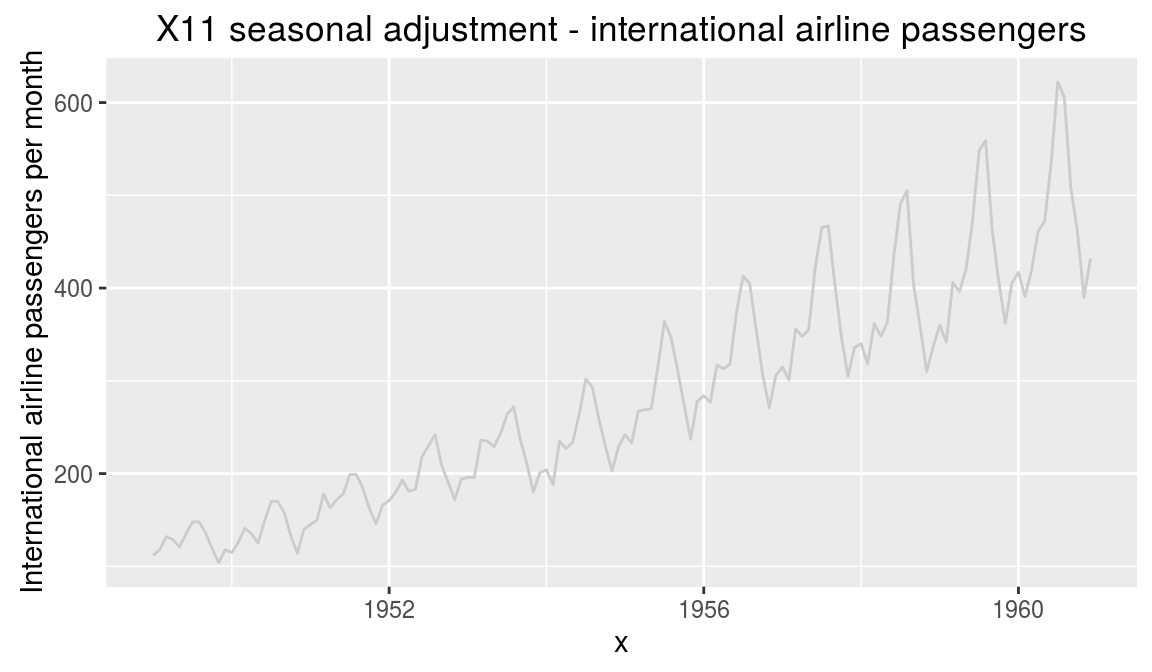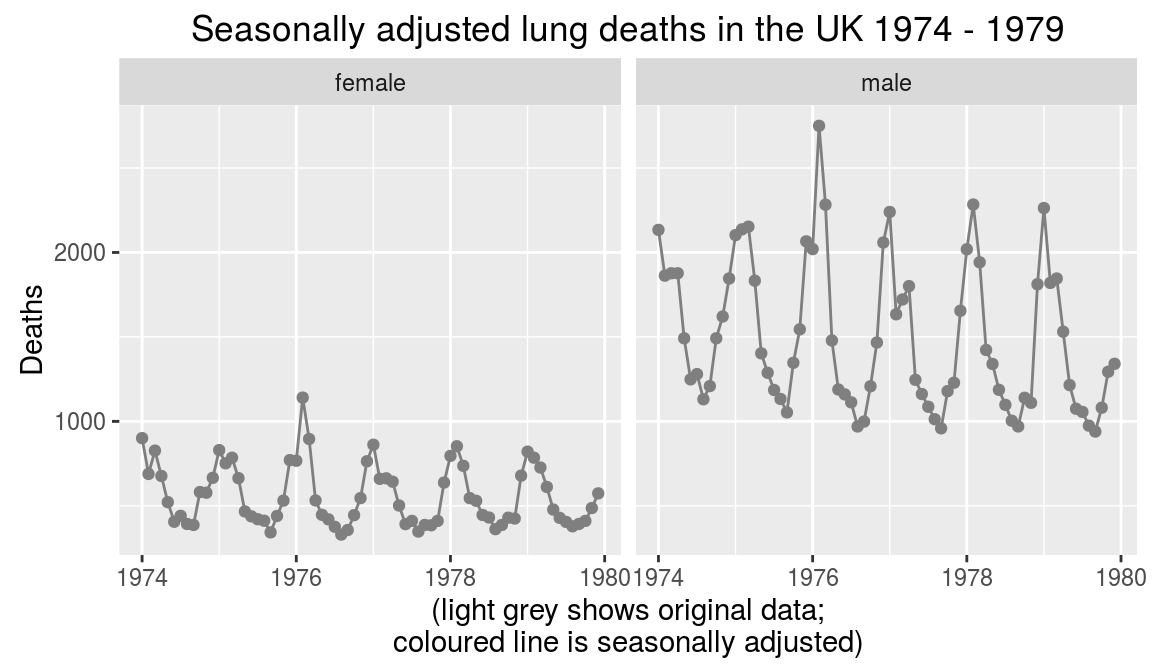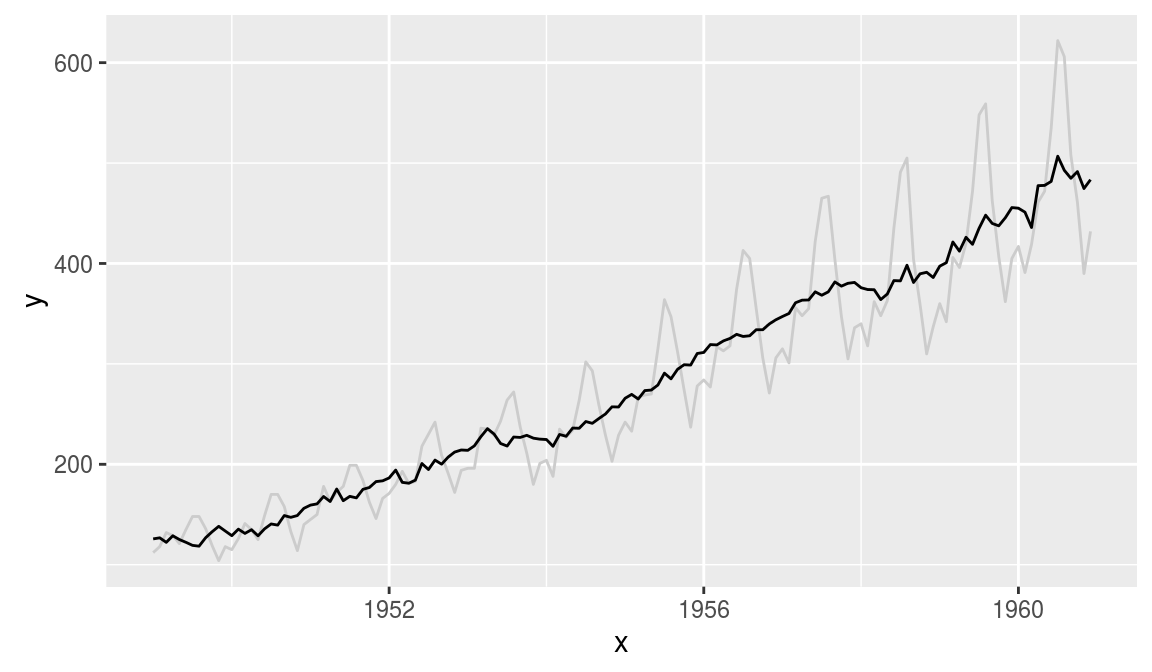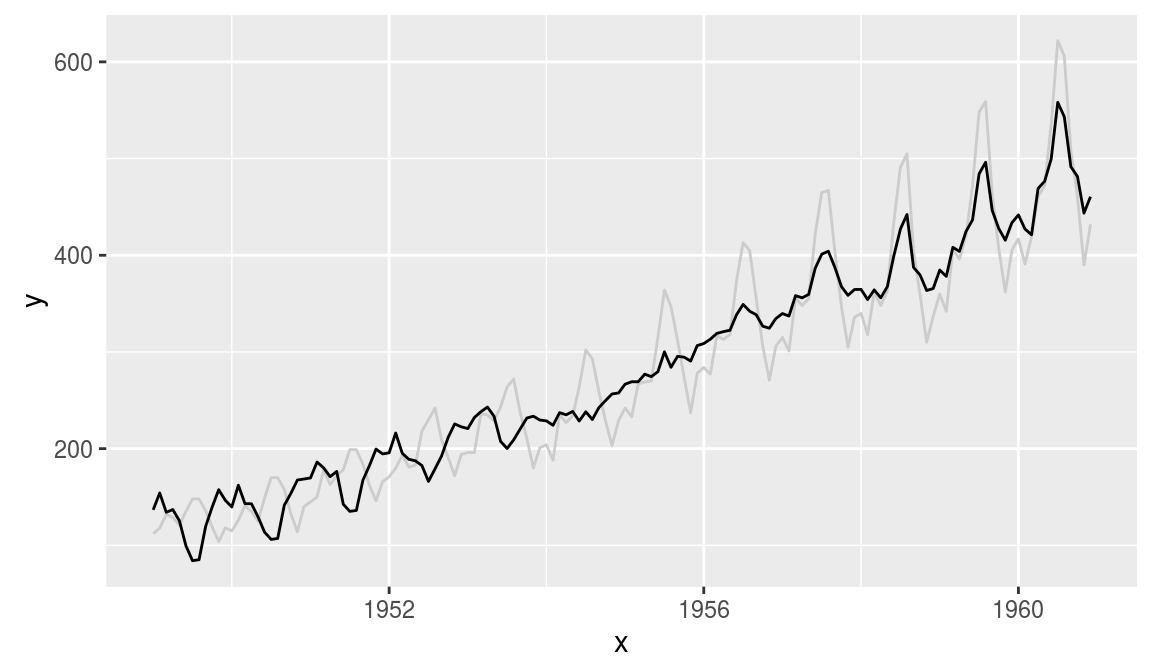ggplot2 now has an official extension mechanism. This means that others can now easily create their own stats, geoms and positions, and provide them in other packages. This should allow the ggplot2 community to flourish, even as less development work happens in ggplot2 itself. This page showcases these extensions.

### ggseas

https://github.com/ellisp/ggseas

Seasonal adjustment on the fly extension for ggplot2. Convenience functions that let you easily do seasonal adjustment on the fly with ggplot. Depends on the `seasonal` package to give you access to X13-SEATS-ARIMA.

``````# Example from https://github.com/ellisp/ggseas
library(ggplot2)
library(ggnet)
library(ggseas)``````

#### Usage

So far there are three types of seasonal adjustment possible

##### X13-SEATS-ARIMA
``````# make demo data
ap_df <- data.frame(
x = as.numeric(time(AirPassengers)),
y = as.numeric(AirPassengers)
)

# SEATS with defaults
ggplot(ap_df, aes(x = x, y = y)) +
geom_line(colour = "grey80") +
stat_seas(start = c(1949, 1), frequency = 12) +
ggtitle("SEATS seasonal adjustment - international airline passengers") +
ylab("International airline passengers per month")````````````# X11 with no outlier treatment
ggplot(ap_df, aes(x = x, y = y)) +
geom_line(colour = "grey80") +
stat_seas(start = c(1949, 1), frequency = 12, x13_params = list(x11 = "", outlier = NULL)) +
ggtitle("X11 seasonal adjustment - international airline passengers") +
ylab("International airline passengers per month")````````````ggplot(ldeaths_df, aes(x = YearMon, y = deaths, colour = sex)) +
geom_point(colour = "grey50") +
geom_line(colour = "grey50") +
facet_wrap(~sex) +
stat_seas(start = c(1974, 1), frequency = 12, size = 2) +
ggtitle("Seasonally adjusted lung deaths in the UK 1974 - 1979") +
ylab("Deaths") +
xlab("(light grey shows original data;\ncoloured line is seasonally adjusted)") +
theme(legend.position = "none")``````##### STL (LOESS-based decomposition)
``````# periodic if fixed seasonality; doesn't work well:
ggplot(ap_df, aes(x = x, y = y)) +
geom_line(colour = "grey80") +
stat_stl(frequency = 12, s.window = "periodic")````````````# seasonality varies a bit over time, works better:
ggplot(ap_df, aes(x = x, y = y)) +
geom_line(colour = "grey80") +
stat_stl(frequency = 12, s.window = 7)``````#### Classical decomposition

``````# default additive decomposition (doesn't work well in this case!):
ggplot(ap_df, aes(x = x, y = y)) +
geom_line(colour = "grey80") +
stat_decomp(frequency = 12)````````````# multiplicative decomposition, more appropriate:
ggplot(ap_df, aes(x = x, y = y)) +
geom_line(colour = "grey80") +
stat_decomp(frequency = 12, type = "multiplicative")``````